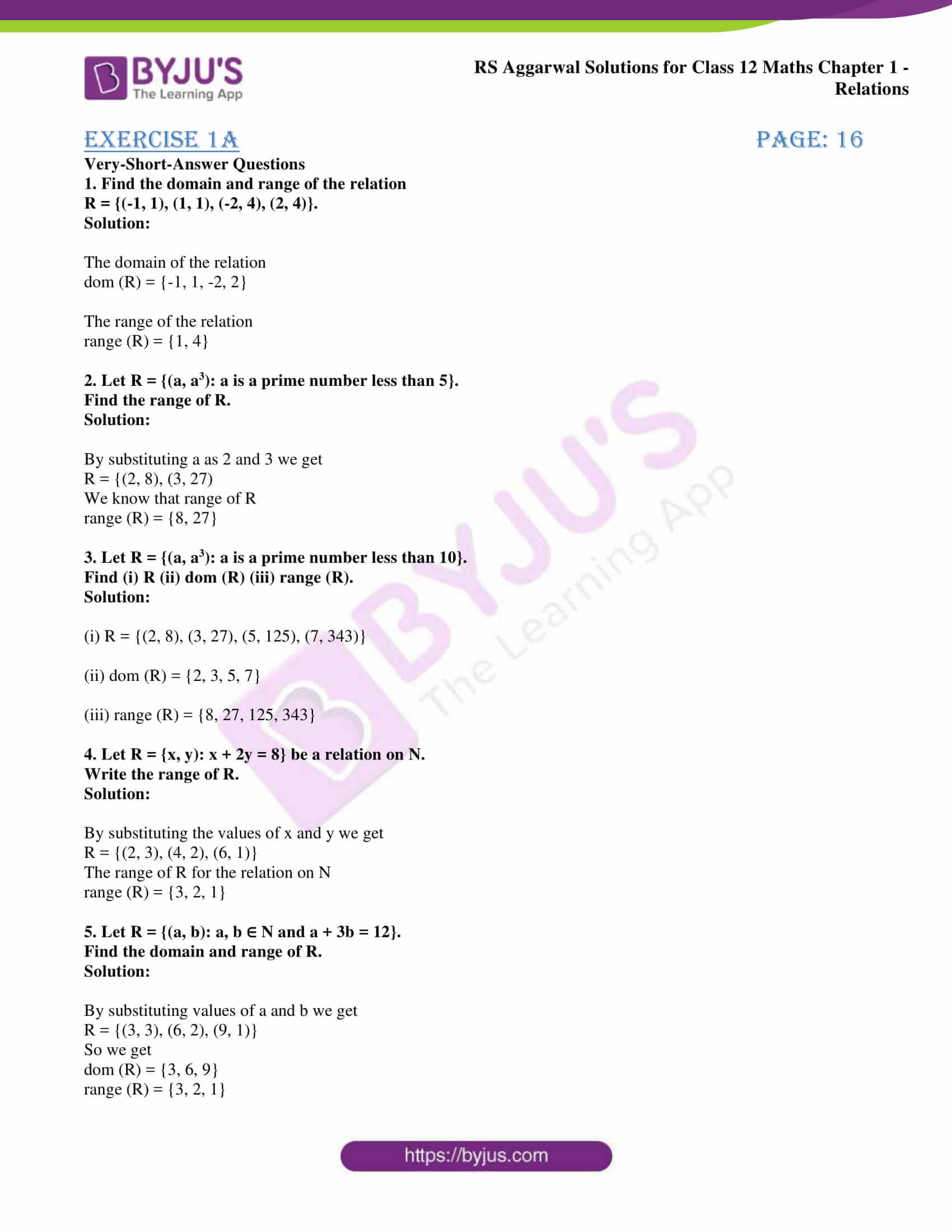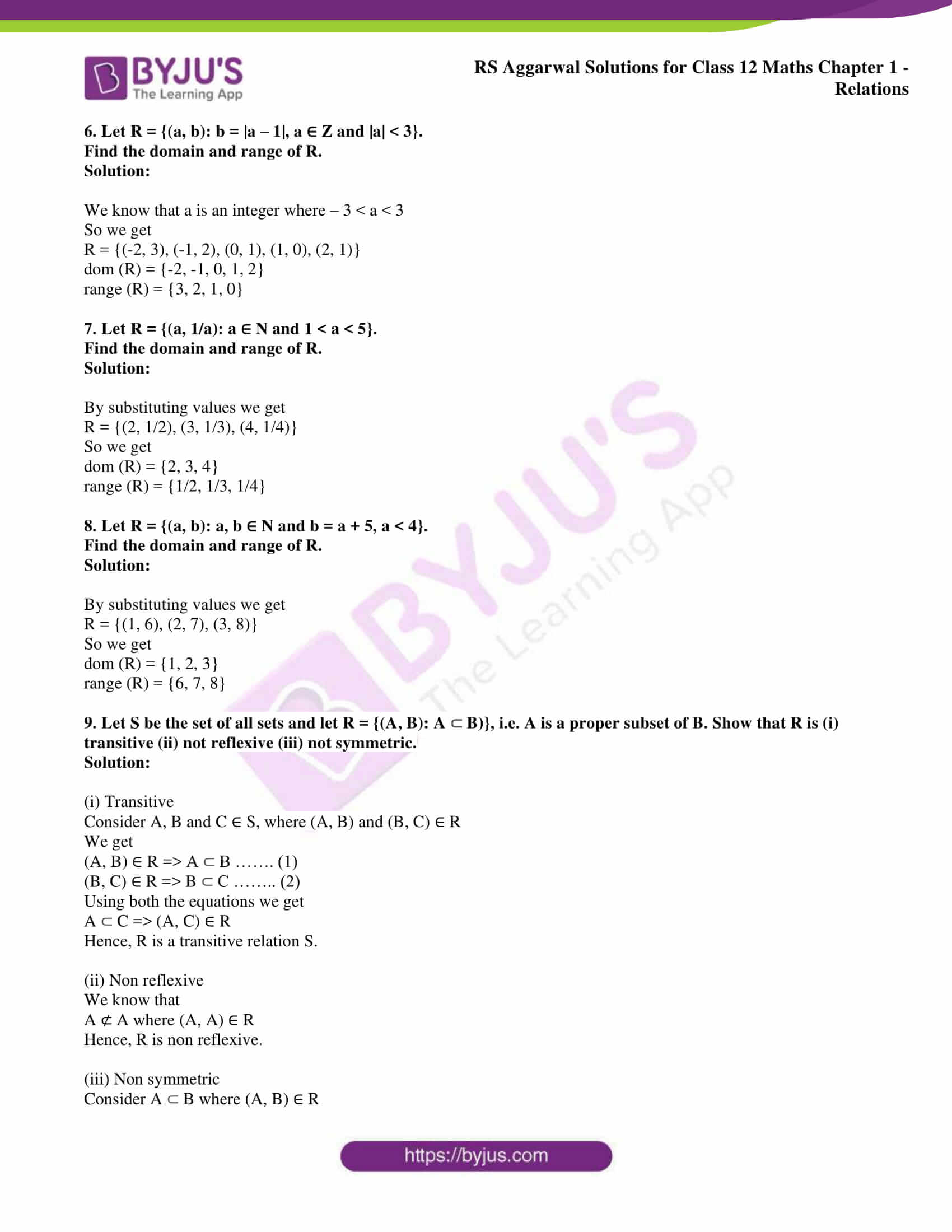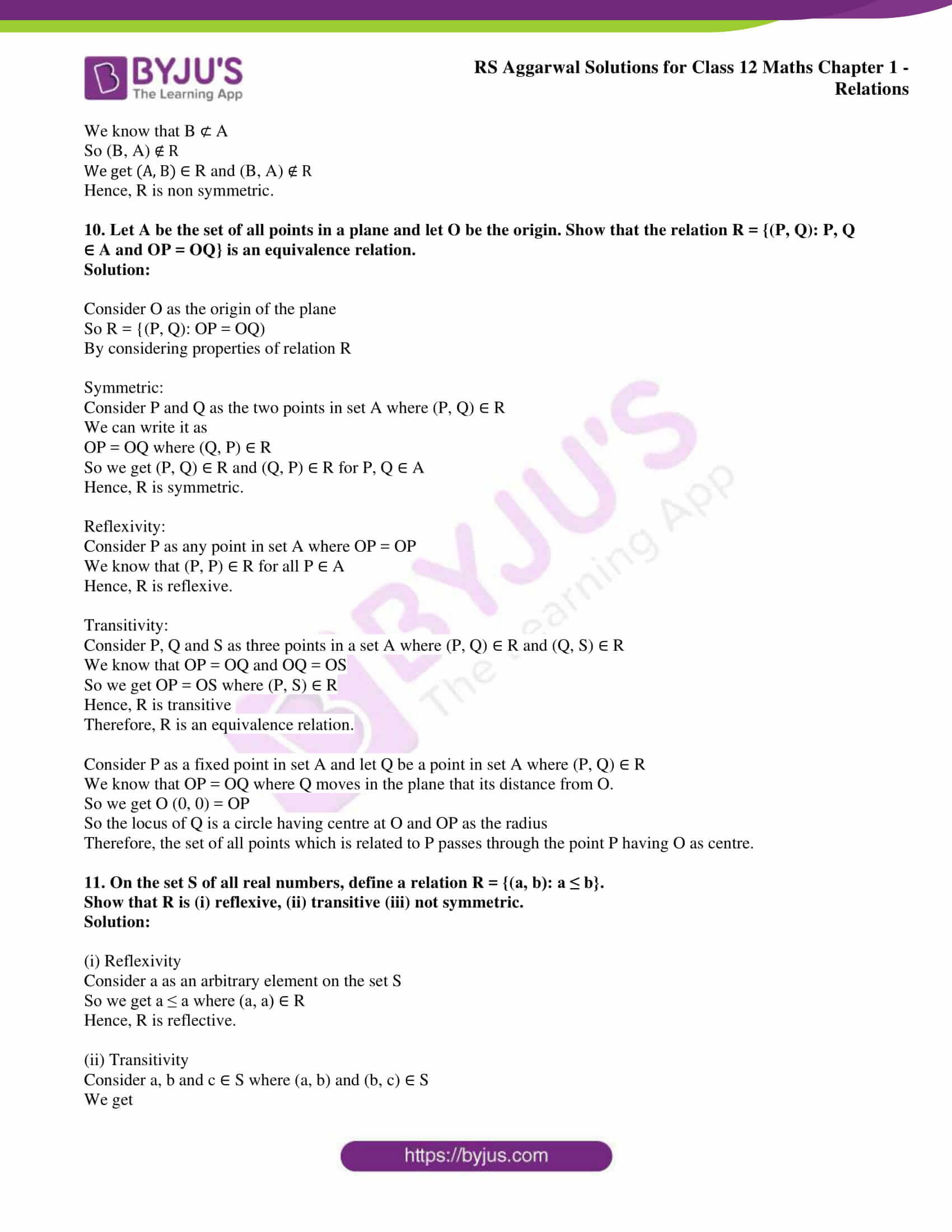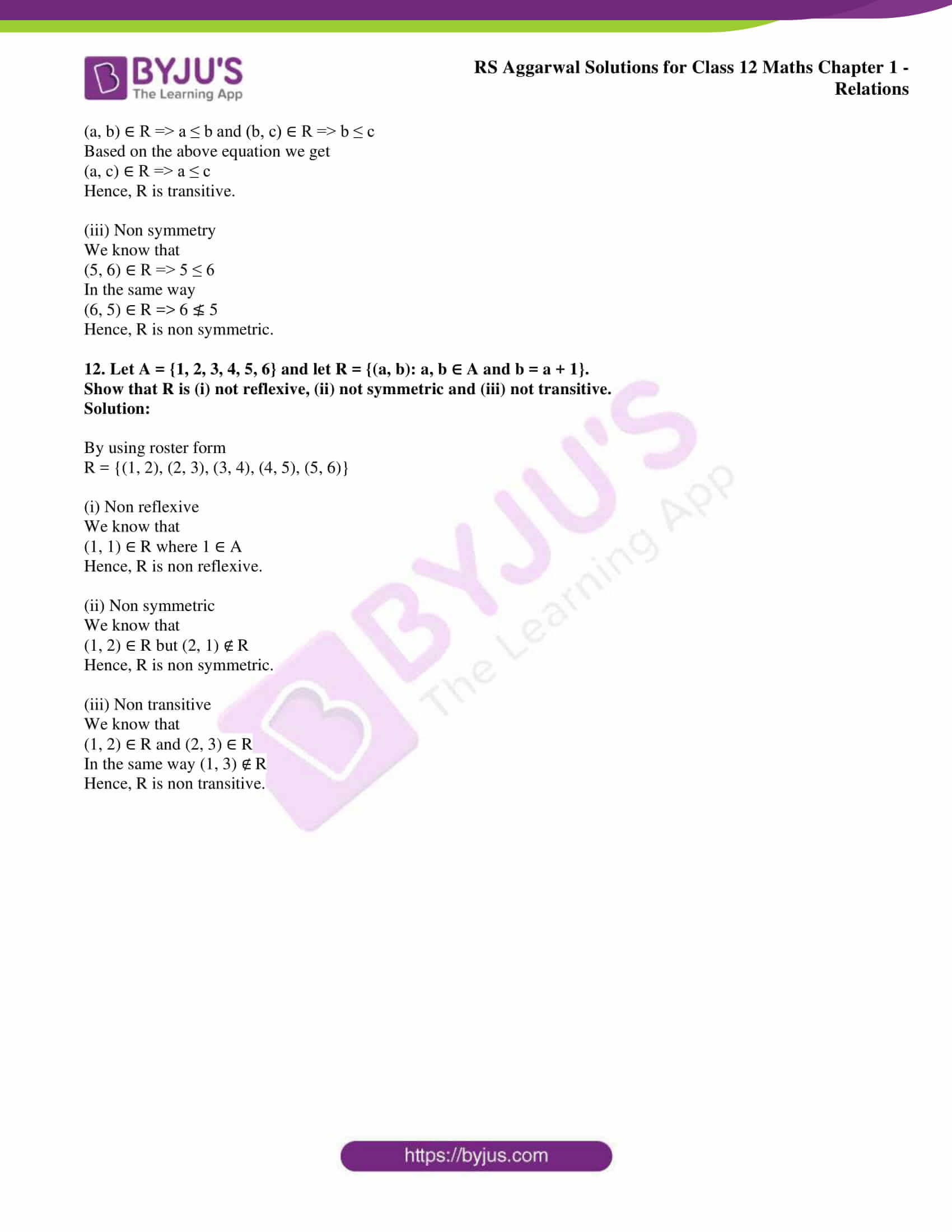# RS Aggarwal Solutions for Class 12 Maths Chapter 1: Relations Exercise 1A

In this exercise, students will mainly get conceptual knowledge about relations. The problems help students understand the method of determining domain and range of a given set of relations. The solutions for the exercise wise problems are prepared by experts at BYJU’S in the best possible way understandable by students. To boost exam preparation of students RS Aggarwal Solutions Class 12 Maths Chapter 1 Relations Exercise 1A PDF is available here for free download.

## RS Aggarwal Solutions for Class 12 Maths Chapter 1: Relations Exercise 1A Download PDF### Access other exercise solutions of Class 12 Maths Chapter 1: Relations

Exercise 1B Solutions

### Access RS Aggarwal Solutions for Class 12 Maths Chapter 1: Relations Exercise 1A

1. Find the domain and range of the relation

R = {(-1, 1), (1, 1), (-2, 4), (2, 4)}.

Solution:

The domain of the relation

dom (R) = {-1, 1, -2, 2}

The range of the relation

range (R) = {1, 4}

2. Let R = {(a, a3): a is a prime number less than 5}.

Find the range of R.

Solution:

By substituting a as 2 and 3 we get

R = {(2, 8), (3, 27)

We know that range of R

range (R) = {8, 27}

3. Let R = {(a, a3): a is a prime number less than 10}.

Find (i) R (ii) dom (R) (iii) range (R).

Solution:

(i) R = {(2, 8), (3, 27), (5, 125), (7, 343)}

(ii) dom (R) = {2, 3, 5, 7}

(iii) range (R) = {8, 27, 125, 343}

4. Let R = {x, y): x + 2y = 8} be a relation on N.

Write the range of R.

Solution:

By substituting the values of x and y we get

R = {(2, 3), (4, 2), (6, 1)}

The range of R for the relation on N

range (R) = {3, 2, 1}

5. Let R = {(a, b): a, b ∈ N and a + 3b = 12}.

Find the domain and range of R.

Solution:

By substituting values of a and b we get

R = {(3, 3), (6, 2), (9, 1)}

So we get

dom (R) = {3, 6, 9}

range (R) = {3, 2, 1}

6. Let R = {(a, b): b = |a – 1|, a ∈ Z and |a| < 3}.

Find the domain and range of R.

Solution:

We know that a is an integer where – 3 < a < 3

So we get

R = {(-2, 3), (-1, 2), (0, 1), (1, 0), (2, 1)}

dom (R) = {-2, -1, 0, 1, 2}

range (R) = {3, 2, 1, 0}

7. Let R = {(a, 1/a): a ∈ N and 1 < a < 5}.

Find the domain and range of R.

Solution:

By substituting values we get

R = {(2, 1/2), (3, 1/3), (4, 1/4)}

So we get

dom (R) = {2, 3, 4}

range (R) = {1/2, 1/3, 1/4}

8. Let R = {(a, b): a, b ∈ N and b = a + 5, a < 4}.

Find the domain and range of R.

Solution:

By substituting values we get

R = {(1, 6), (2, 7), (3, 8)}

So we get

dom (R) = {1, 2, 3}

range (R) = {6, 7, 8}

9. Let S be the set of all sets and let R = {(A, B): A ⊂ B)}, i.e. A is a proper subset of B. Show that R is (i) transitive (ii) not reflexive (iii) not symmetric.

Solution:

(i) Transitive

Consider A, B and C ∈ S, where (A, B) and (B, C) ∈ R

We get

(A, B) ∈ R => A ⊂ B ……. (1)

(B, C) ∈ R => B ⊂ C …….. (2)

Using both the equations we get

A ⊂ C => (A, C) ∈ R

Hence, R is a transitive relation S.

(ii) Non reflexive

We know that

A ⊄ A where (A, A) ∈ R

Hence, R is non reflexive.

(iii) Non symmetric

Consider A ⊂ B where (A, B) ∈ R

We know that B ⊄ A

So (B, A) ∉ R

We get (A, B) ∈ R and (B, A) ∉ R

Hence, R is non symmetric.

10. Let A be the set of all points in a plane and let O be the origin. Show that the relation R = {(P, Q): P, Q ∈ A and OP = OQ} is an equivalence relation.

Solution:

Consider O as the origin of the plane

So R = {(P, Q): OP = OQ)

By considering properties of relation R

Symmetric:

Consider P and Q as the two points in set A where (P, Q) ∈ R

We can write it as

OP = OQ where (Q, P) ∈ R

So we get (P, Q) ∈ R and (Q, P) ∈ R for P, Q ∈ A

Hence, R is symmetric.

Reflexivity:

Consider P as any point in set A where OP = OP

We know that (P, P) ∈ R for all P ∈ A

Hence, R is reflexive.

Transitivity:

Consider P, Q and S as three points in a set A where (P, Q) ∈ R and (Q, S) ∈ R

We know that OP = OQ and OQ = OS

So we get OP = OS where (P, S) ∈ R

Hence, R is transitive

Therefore, R is an equivalence relation.

Consider P as a fixed point in set A and let Q be a point in set A where (P, Q) ∈ R

We know that OP = OQ where Q moves in the plane that its distance from O.

So we get O (0, 0) = OP

So the locus of Q is a circle having centre at O and OP as the radius

Therefore, the set of all points which is related to P passes through the point P having O as centre.

11. On the set S of all real numbers, define a relation R = {(a, b): a ≤ b}.

Show that R is (i) reflexive, (ii) transitive (iii) not symmetric.

Solution:

(i) Reflexivity

Consider a as an arbitrary element on the set S

So we get a ≤ a where (a, a) ∈ R

Hence, R is reflective.

(ii) Transitivity

Consider a, b and c ∈ S where (a, b) and (b, c) ∈ S

We get

(a, b) ∈ R => a ≤ b and (b, c) ∈ R => b ≤ c

Based on the above equation we get

(a, c) ∈ R => a ≤ c

Hence, R is transitive.

(iii) Non symmetry

We know that

(5, 6) ∈ R => 5 ≤ 6

In the same way

(6, 5) ∈ R => 6 ≰ 5

Hence, R is non symmetric.

12. Let A = {1, 2, 3, 4, 5, 6} and let R = {(a, b): a, b ∈ A and b = a + 1}.

Show that R is (i) not reflexive, (ii) not symmetric and (iii) not transitive.

Solution:

By using roster form

R = {(1, 2), (2, 3), (3, 4), (4, 5), (5, 6)}

(i) Non reflexive

We know that

(1, 1) ∈ R where 1 ∈ A

Hence, R is non reflexive.

(ii) Non symmetric

We know that

(1, 2) ∈ R but (2, 1) ∉ R

Hence, R is non symmetric.

(iii) Non transitive

We know that

(1, 2) ∈ R and (2, 3) ∈ R

In the same way (1, 3) ∉ R

Hence, R is non transitive.